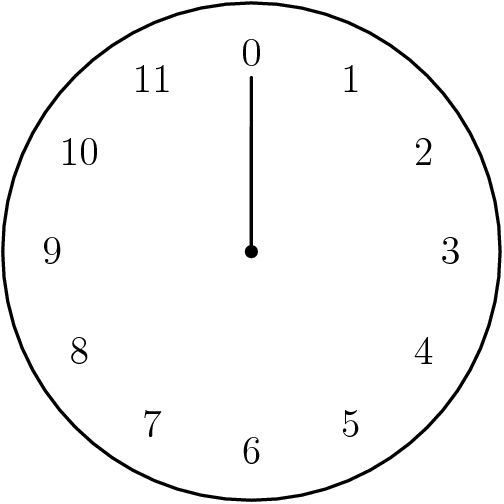A# modular arithmeticModular arithmetic is a special type of arithmetic, involving only integers, in which numbers wrap around upon reaching a certain value, known as the modulus. The principle of it can be understood in terms of a clock face, numbered in the usual way except that the 12 is replaced by 0. As the hour hand sweeps around the sequence of numbers it passes is:

0, 1, 2, 3, 4, 5, 6, 7, 8, 9, 10, 11, 0, 1, 2, 3, 4, 5,...

This is how counting is done in modulo 12.

Similarly, in modulo 7 the sequence would be:

0, 1, 2, 3, 4, 5, 6, 0, 1, 2, 3, 4, 5, 6, 0, ...

Modulo 7 arithmetic describes the repeating sequence of seven notes, from A to G, used in music:

A, B, C, D, E, F, G, A, B, C, D, E, F, G, A, ...

in which every eighth note returns to the same letter (but shifted by an octave).

The term residue is given to any of the set of numbers that repeats. In the case of modulo 7, the residues are 0, 1, 2, 3, 4, 5, and 6. In general the residues in modulo n are 0,1, 2, ..., n - 1.

The term congruent is applied to numbers that are equivalent for a given modulo. For example, in modulo 7, the numbers 2 and 9 are congruent, because 2 + 7 = 9. Similarly, 2 and 16 are congruent because 2 + (2 × 7) = 16.